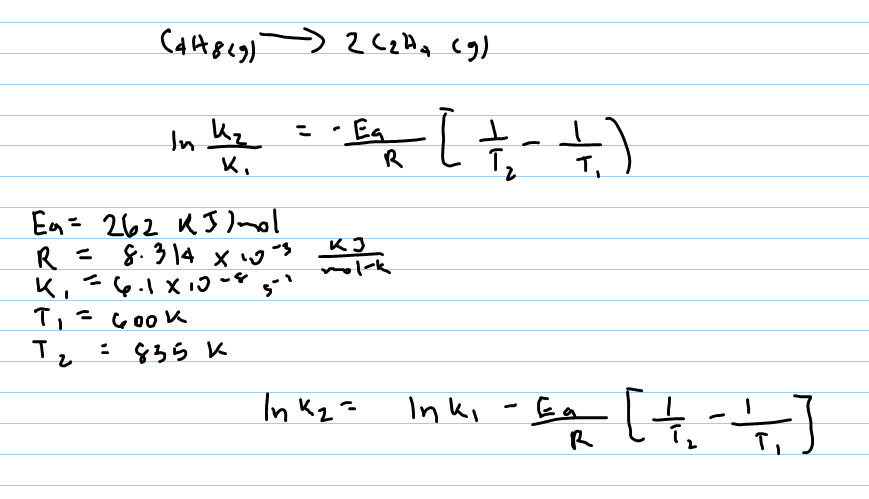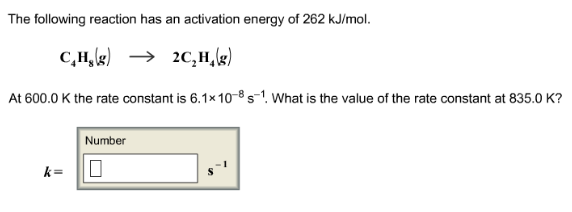# Problem: The following reaction has an activation energy of 262 kJ/mol. C4H8(g) → 2C4H4(g) At 600.0K the rate constant is 6.1 x 10-8 s-1. What is the value of the rate constant at 835.0K?

###### FREE Expert Solution85% (63 ratings)###### Problem Details

The following reaction has an activation energy of 262 kJ/mol. C4H8(g) → 2C4H4(g) At 600.0K the rate constant is 6.1 x 10-8 s-1. What is the value of the rate constant at 835.0K?